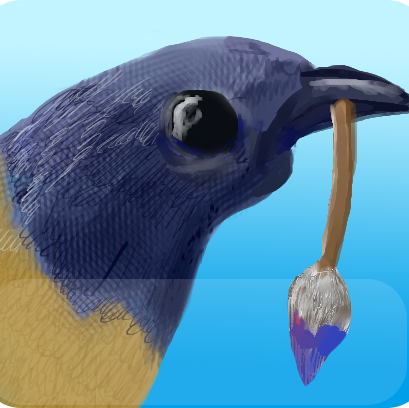# artreepie, a procedural art botLast week, I stumbled upon a great stackexchange discussion on procedural art . The idea was to come up with three code snippets that would be used to generate an image. More specifically, each snippet – one for each color channel (R, G, B) – is a function that, given a pixel coordinate, returns its intensity. Each snippet should fit in a single tweet.

This very interesting exercise inspired me into creating @artreepie . artreepie is a Twitter bot that captures mentions to him containing code snippets and generates art pieces. The three pieces of code sent to artreepie from a user are used to build the image, which is sent back to the user by the bot.

## Implementation

artreepie uses a modified version of twik , a tiny lisp-like language, in its rendering engine. The code snippet to be tweeted to artreepie may use a number of provided variables and functions:

### Variables

Name Description
i Column number of pixel being calculated (starting from zero)
j Row of pixel being calculated (starting from zero)
w Image width in pixels (currently 1024)
h Image height in pixels (currently 1024)

### Functions

Name Syntax Example
If if (if true 1 0)
And and (and true false)
Or or (or true false)
Sine sin (sin 1.0)
Cosine cos (cos 1.0)
Bitwise And & (& 1 2 3)
Bitwise Or | (| 1 2 3 )
Modulo % (% 10 2)
Random [0.0, 1.0) rnd (rnd)
Square Root sqrt (sqrt 16)
Square sq (sq 10)
Less Than < (lt 1 4)
Greater than > (gt 1 4)
Equals == (== 1 1)
Not equal != (!= 1 2)

## Ok, I am ready to use it

In order to use artreepie all you need is a Twitter account. Tweets are processed in the order they were posted: the first tweet will be used for the red channel, the second for the green channel and the third for the blue channel.

This image was generated with the following tweets:

``````@artreepie (& i j)
@artreepie (% (+ i j) 255)
@artreepie (& i j)``````

In case of errors during the computation of the image – e.g. division by zero – the whole image is silently discarded. For testing different expressions before submitting it to artreepie, you may use a command line version of the bot available in the project page on Github .

## Some generated images

Here are some example images, listed in the stackexchange thread, written using twik:

``````@artreepie (if (and (!= i 0) (!= j 0)) (& (% i j) (% j i)) 0)
@artreepie (if (and (!= i 0) (!= j 0)) (+ (% i j) (% j i)) 0)
@artreepie (if (and (!= i 0) (!= j 0)) (| (% i j) (% j i)) 0)``````
See the result .

``````@artreepie (var s (/ 3.0 (+ j 99))) (* (+ (% (+ (* j s) (* s (+ i w))) 2) (% (+ (* j s) (* s (- (* w 2) i))) 2)) 127)
@artreepie (var s (/ 3.0 (+ j 99))) (* (+ (% (+ (* j s) (* s (+ i w))) 2) (% (+ (* j s) (* s (- (* w 2) i))) 2)) 127)
@artreepie (var s (/ 3.0 (+ j 99))) (* (+ (% (+ (* j s) (* s (+ i w))) 2) (% (+ (* j s) (* s (- (* w 2) i))) 2)) 127)``````
See the result .